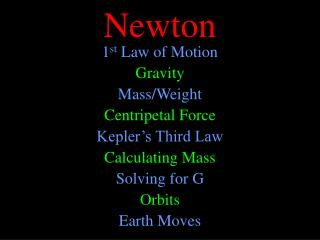DownloadDownload PresentationNewton

# Newton

Download Presentation## Newton

- - - - - - - - - - - - - - - - - - - - - - - - - - - E N D - - - - - - - - - - - - - - - - - - - - - - - - - - -
##### Presentation Transcript

1. Newton 1st Law of Motion Gravity Mass/Weight Centripetal Force Kepler’s Third Law Calculating Mass Solving for G Orbits Earth Moves

2. Newton’s 1st An object at rest remains at rest, and an object in motion continues in a straight line unless acted on by an outside force.

3. Newton’s 1st An object at rest remains at rest unless acted on by an outside force.

4. Newton’s 1st An object in motion continues in a straight line unless acted on by an outside force.

5. Newton 1st Law of Motion Gravity Mass/Weight Centripetal Force Kepler’s Third Law Calculating Mass Solving for G Orbits Earth Moves

6. Gravity -G M m r2 Fg =

7. Gravity -G M m r2 Fg = Attractive Force

8. Gravity -G M m r2 Fg = Universal Gravitational Constant

9. Gravity -G M m r2 Fg = Masses of Two Objects

10. Gravity -G M m r2 Fg = Distance Between Centers

11. -G M m r2 Fg = Gravity 8 X ¤ 64 X

12. Newton 1st Law of Motion Gravity Mass/Weight Centripetal Force Kepler’s Third Law Calculating Mass Solving for G Orbits Earth Moves

13. Mass / Weight Mass = Quantity of Matter Which has more matter, a pound of lead or a pound of feathers? The pound of feathers is bigger, but that’s a different question. The pound of lead is denser, but that’s a different question. If they are both on Earth, they have the same mass. A pound of feathers on the moon has more mass than a pound of lead on Earth. If I take a pound of lead to the moon, it will weigh less, but the mass will still be the same. Weight = Force of Gravity Holding it to Surface kilogram = measure of mass pound = measure of force

14. Mass / Weight With a mass of 68 kg, I weigh 150 lbs on Earth. The moon’s gravity is weaker. I would only weigh 31.5 lbs there. On Mars, I would weigh 67.5 lbs.

15. Newton 1st Law of Motion Gravity Mass/Weight Centripetal Force Kepler’s Third Law Calculating Mass Solving for G Orbits Earth Moves

16. Gravity is a Centripetal Force. Any force that is directed toward the center of motion. A Ball on a String A Car on a Curved Road

17. "

18. Fc= -m v2 r

19. -G M m r2 Fg Fc= = Centripetal Force -m v2 r =

20. Centripetal Force -m v2 r -G M m r2 =

21. Centripetal Force -m v2 r -G M m r2 =

22. 2 p r P Circular Orbit v = Centripetal Force v2 r G M r2 =

23. Centripetal Force 22p2r2 P2 Circular Orbit v2= v2 r G M r2 =

24. Centripetal Force 4p2r2 P2r G M r2 =

25. P2 P2 Centripetal Force 4p2r P2 G M r2 =

26. r2 r2 Centripetal Force G M r2 4p2r = P2

27. 1 G M 1 G M Centripetal Force 4p2r3 = G M P2

28. Centripetal Force Circular Orbit r = a 4p2 G M r3 = P2

29. Centripetal Force Circular Orbit r = a 4p2 G M a3 = P2

30. Kepler’s Third Law P2 = ka3 4p2 G M a3 = P2 ¤

31. Newton 1st Law of Motion Gravity Mass/Weight Centripetal Force Kepler’s Third Law Calculating Mass Solving for G Orbits Earth Moves

32. M M Finding Mass 4p2 G M a3 = P2

33. 1 P2 1 P2 Finding Mass 4p2 G a3 = P2 M

34. Finding Mass One problem remains. a3 P2 4p2 G = M

35. Finding Mass One problem remains. a3 P2 4p2 G = M

36. m m -GMÅm RÅ2 -GMÅm RÅ2 -GMm D2 F = = + Mass of Earth Phillip von Jolly M

37. m m Mass of Earth Phillip von Jolly M -GMÅm RÅ2 -GMm D2 -GMÅm RÅ2 F = = +

38. -GMÅm RÅ2 -GMm D2 -GMÅm RÅ2 F = = + Mass of Earth Phillip von Jolly m m n M -GMÅn RÅ2 +

39. Mass of Earth -GMÅm RÅ2 -GMm D2 -GMÅm RÅ2 -GMÅn RÅ2 F = = + +

40. -GMm D2 -GMÅn RÅ2 = RÅ2 n RÅ2 n Mm D2 MÅn RÅ2 = ( ) m RÅ2 n D M = MÅ Mass of Earth

41. Finding Mass One problem remains. a3 P2 4p2 G = MÅ ( (

42. Newton 1st Law of Motion Gravity Mass/Weight Centripetal Force Kepler’s Third Law Calculating Mass Solving for G Orbits Earth Moves

43. Orbits Å Apogee Perigee Circular

44. Orbits Å Apogee Perigee

45. Å Å Transfer Orbits Å ¤

46. Newton 1st Law of Motion Gravity Mass/Weight Centripetal Force Kepler’s Third Law Calculating Mass Solving for G Orbits Earth Moves

47. Proof of Earth’s Motion Rotation Revolution

48. Proof of Earth’s Revolution What would satisfy Aristotle? Parallax

49. Parallax Å ¤ Å